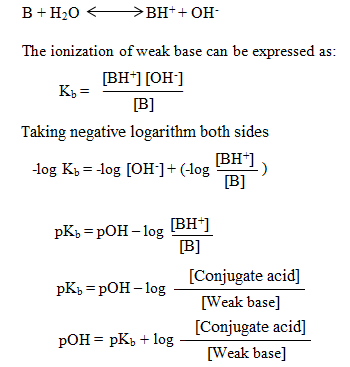# Derive an equation similar to the Henderson–Hasselbalch equation for a buffer composed of a weak base and its conjugate acid. Instead of relating pH to pKa and the relative concentrations of an acid and its conjugate base (as the Henderson– Hasselbalch equation does), the equation should relate pOH to pKa and the relative concentrations of a base and its conjugate acid.

Question
13 views

Derive an equation similar to the Henderson–Hasselbalch equation for a buffer composed of a weak base and its conjugate acid. Instead of relating pH to pKa and the relative concentrations of an acid and its conjugate base (as the Henderson– Hasselbalch equation does), the equation should relate pOH to pKa and the relative concentrations of a base and its conjugate acid.

check_circle

Step 1

Henderson Hasselbalch equation is one that relates pH of buffer with ionization constants of the weak electrolyte.

For a buffer consisting of weak acid and its conjugate base, pH of buffer is expressed as :

pH = pKa + log[Conjugate base]/[Weak Acid]

Step 2

For a buffer consisting of weak base and its salt with strong acid, the pH is expressed as:

pOH = pKb + log [Conjugate acid]/[Weak base]

For a weak base, B, which forms  BH+ as its conjugate acid, the above expression can be derived as:Step 3

Now,  if Kb is ionization constant for weak base and Ka is ionization constant of its conjugate acid,

Ka *Kb = 10-14.

Taking negative logarithm both sides,

-log(Ka *Kb) = -log(10-14)

-log Ka + (-log Kb) = 14

pKa + pKb = 14

So, pKb = 14- pKa

Substituting 'pKb' by '14-pKa' in expression deri...

### Want to see the full answer?

See Solution

#### Want to see this answer and more?

Solutions are written by subject experts who are available 24/7. Questions are typically answered within 1 hour.*

See Solution
*Response times may vary by subject and question.
Tagged in

### Chemistry